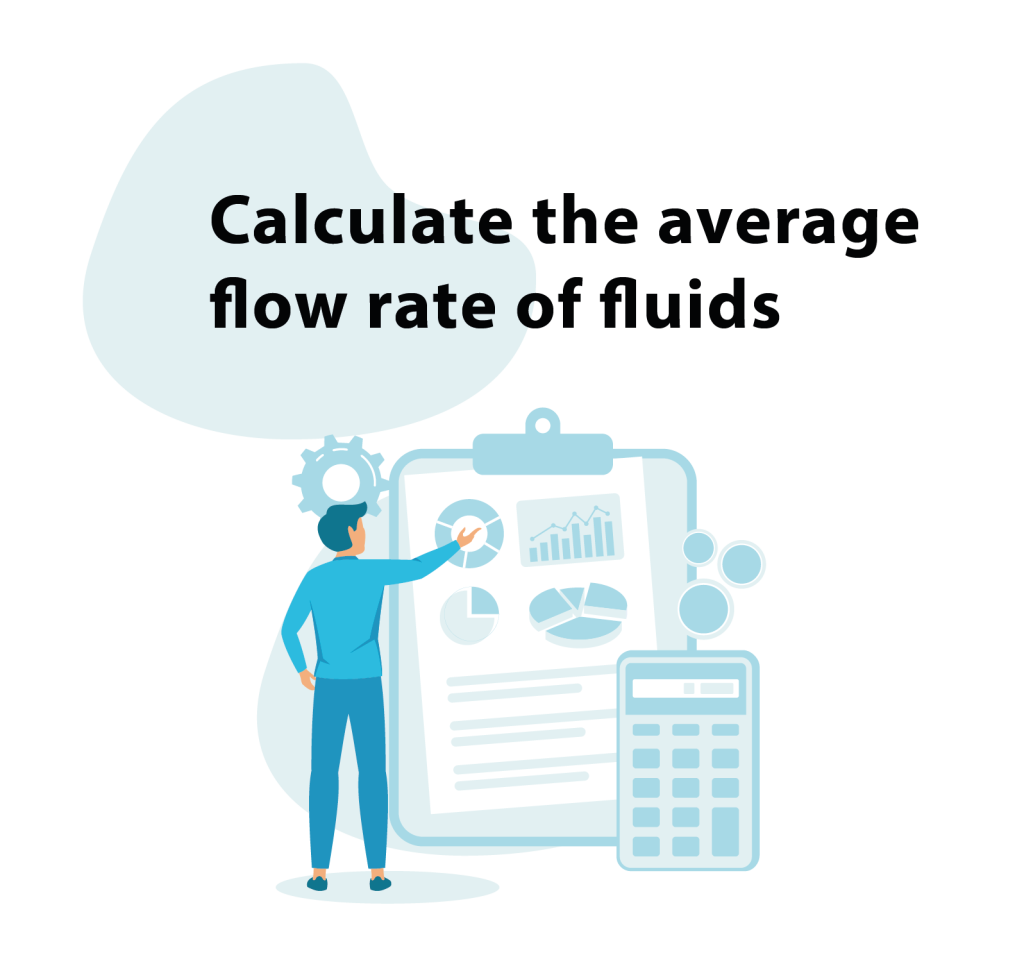• ### Call us# Flow Rate Calculator

With this Flow Rate Calculator, it is possible to easily calculate the average volumetric flow rate of fluids by changing each of the three variables: length, pressure and bore diameter. The effects on the predicted flow rate are then given in three graphs, where in turn two of the variables are kept constant and the flow rate is plotted against a range of values of the third.

### How to Use:

To begin calculating, enter your figures in the boxes below. If a value is not available
for any one of the variables, then please leave the field blank and the program will select its own value.